Database Reference FormulasAs we have been discussing, an Excel worksheet maintains its bi-directional connection to PowerOLAP® through the use of database reference formulas. Database references look like formulas, but rather than provide arithmetic calculations, the references (at least in the case of Fact Data cells) refer to individual cells (or Dimension-Member intersection points) within PowerOLAP database Cubes. The database references are similar to Excel formulas that link two or more worksheets within a workbook. However, as you have already seen, PowerOLAP database references provide far more power than Excel formulas, as these key features indicate:

• Database references can be bi-directional. Thus, data can be retrieved from a PowerOLAP database Cube, and data can be entered through a worksheet into a PowerOLAP cube.
• Database reference formulas remain intact in worksheet cells even when data is typed over them. Typed values do not overwrite the references. Only accidental deletion of cell contents overwrites the references. (Note: database reference formulas can be restored easily by copying and pasting the formula from an adjacent cell back into a cleared cell.)
• Database reference formulas can be built using Member names, Alias names, or Excel cell references. The reference may contain one of these formats, or a combination of all three.

The following pages concern key aspects of database reference formulas, including the structure of the references; building a reference formula using the Edit Formula Wizard; and modifying Edit Formula text.

The Structure of Database References

The following figure illustrates an example of a PowerOLAP database reference we have already discussed, containing OLAPReadWrite formulas. Clicking on a Fact Data cell (e.g., cell B9 of the example worksheet) shows the database reference that appears in the Excel formula bar as follows: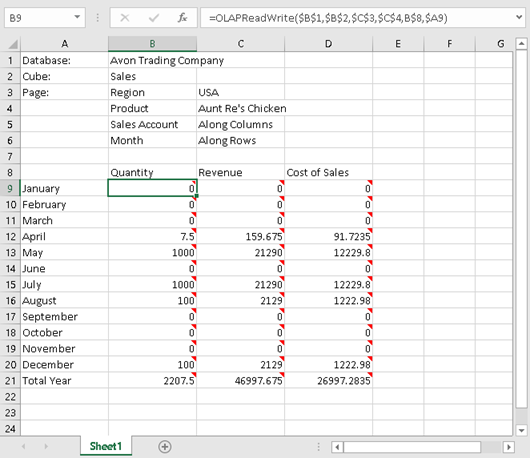As we have seen, OLAPReadWrite is the PowerOLAP database reference formula function that enables values to be read from and written to a Cube. Each of the cell references within the parentheses is called a database reference argument.

The following is a breakdown of the example database reference arguments:

• \$B\$1     PowerOLAP Database Path and Name
• \$B\$2     PowerOLAP Database Cube Name
• \$C\$3     Dimension Region Page Member (USA)
• \$C\$4     Dimension Product Page Member (Aunt Re’s Chicken)
• B\$8      Dimension Sales Account Member (Quantity)
• \$A9      Dimension Month Member (January)

Therefore, the database reference arguments at this moment are accessing the example file Avon Trading Company, Sales cube; for the Region dimension, Member USA; for the Product dimension, Member Aunt Re’s Chicken; for the Sales Account dimension, Member Quantity; for the Month dimension, Member January.

By using cell references, you have the flexibility to change arguments to access different locations within a PowerOLAP database. You could access, for example, different Page members; groups or lists of row and column Dimension Members; or even different Cubes within the database, all by changing the reference arguments.TipYou can quickly identify database references by double-clicking on a worksheet cell: all references to that cell will be highlighted in the worksheet, as in the figure below (e.g., when B9 is double-clicked).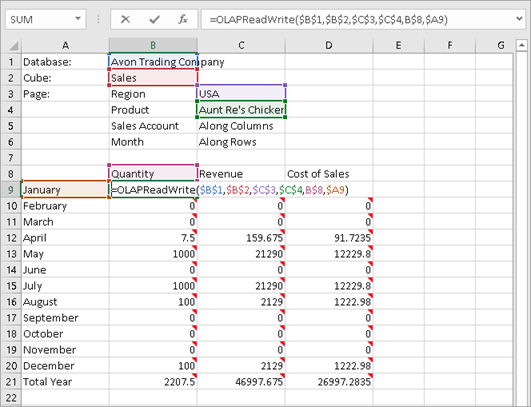Note that the referenced cells (for B9) are highlighted.

Build a Database Reference Formula: Edit Formula WizardEdit Formulain Excel Building a database reference in an Excel worksheet is not a complex operation, even for an introductory user. For one, it is not necessary to write the database references entirely on your own: PowerOLAP® provides an Edit Formula Wizard to assist you with the process of building database reference formulas.

.

As discussed in the previous section, database references are used to maintain the bi-directional connection between Excel worksheets and PowerOLAP databases. Although PowerOLAP® automatically builds database reference formulas when you “Slice” data into an Excel worksheet, you may want to build additional formulas or edit existing formulas within the worksheet. Using the Edit Formula function, you can create a connection to a PowerOLAP database to return a single cell reference in your worksheet instead of an entire ‘sliced’ table. PowerOLAP’s Edit Formula Wizard simplifies the task for you.

The following procedure will demonstrate how to build a database reference formula within an Excel worksheet that is linked to a PowerOLAP database. [In fact, this will involve clearing and restoring data that currently exists in the worksheet, but the procedure is the same if you are beginning in a blank cell.]

1.   Open an Excel worksheet connected to a PowerOLAP database.
We can use the worksheet from the last exercise as an example.

2.   Click on the first cell containing a database reference returning Cube Fact data. In the figure below, the first data cell containing a database reference is B9 (circled).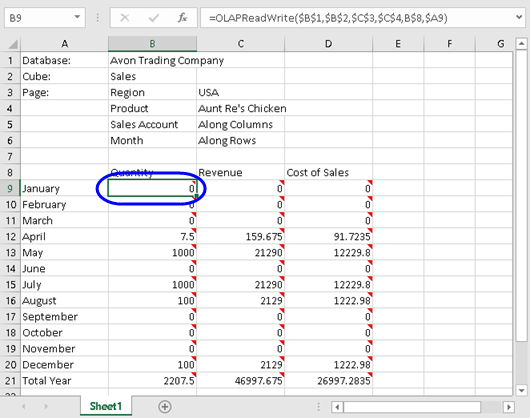3.   Select a range of cells that display Cube data—for example,
B9:D16
(Quantity, January until Cost of Sales, August) in the preceding figure).

4.   Delete the Range of cells that was selected.

5.   Click in the first cell that you want to return Cube data
(in this case, cell B9).

6.   In the Excel ribbon, go to Add-Ins tab, select PowerOLAP and Edit Formula menu command.
The Edit PowerOLAP Formula dialog box is opened: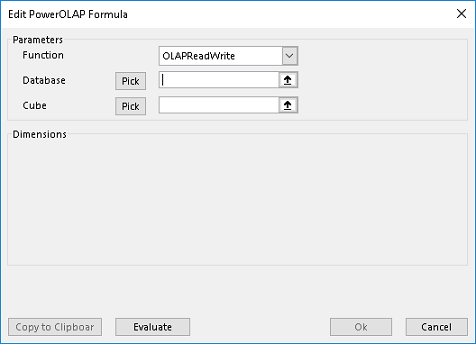The first item in the Edit PowerOLAP Formula dialog box is the Function text box (circled). There are two functions provided by the Edit Formula Wizard from the drop-down menu: OLAPReadWrite, and OLAPRead. The purpose of each is described below:

– Here again we can choose a ReadWrite cell reference in our Excel worksheet.

– The OLAPRead function provides read-only access to a PowerOLAP Cube. Thus, an OLAPRead database reference formula returns data from a Cube into a worksheet cell, but does not allow you to enter data into the worksheet cell and send it back to the database.

The next items in the Edit PowerOLAP Formula dialog box are the Database and Cube text boxes. The Pick buttons each allow you to choose a name from a list of available databases and Cubes. This option is useful when the database and Cube names that you want to access do not appear in your Excel worksheet.

Next step is to pick a database and Cube.

7.   Click the Pick button next to the Database text box.
In the Select Database dialog box that appears, you are given a choice of available databases that can be connected to this worksheet.
Make a selection (in the example, …Avon Trading Company.olp), then click on OK.

8.   Do likewise after clicking the Pick button next to the Cube text box (e.g., pick Sales).

Note that the Dimensions section appears, and the Wizard has “intuitively entered the last two Members. In the example, circled below, Quantity has been entered for the Sales Account dimension, and January for Month. [This occurs because the cell occurs within a known data range—if this were a blank cell, say F9, the dialog box would be slightly different.]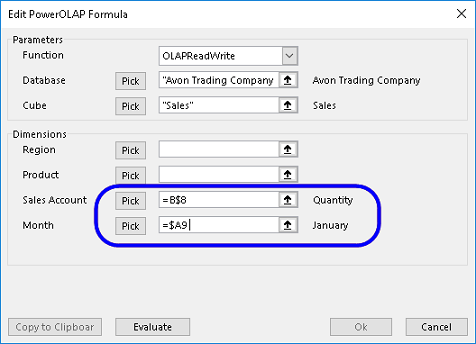9.   Next to Region, click on the Pick button.
The Select a Member dialog box appears—use this dialog box to pick a Member (e.g., USA). Click OK.

10. Next to Product, also click on the Pick button.
Select the desired Member (e.g., Aunt Re’s Chicken). Click OK.

11. If these are correct references for the cell, click OK; otherwise you would use the Pick button to select remaining members.

The following figure illustrates an example of a completed Edit PowerOLAP Formula dialog box: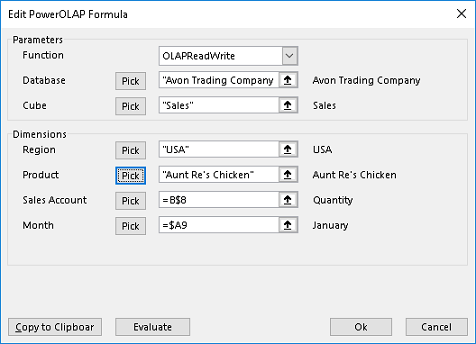12. Click OK to close the dialog box and return to the worksheet.
You will see #RECALC in the cell, so click F9 to recalculate.
The Fact Data value that you specified in the previous steps appears.

13. You can now copy the cell, or “extend the series” to the relative range, e.g., copy B9 across to D9, and then down to D16. This is because PowerOLAP maintains this function in Excel (another example of how PowerOLAP enables users to “work with Excel the way they always do”).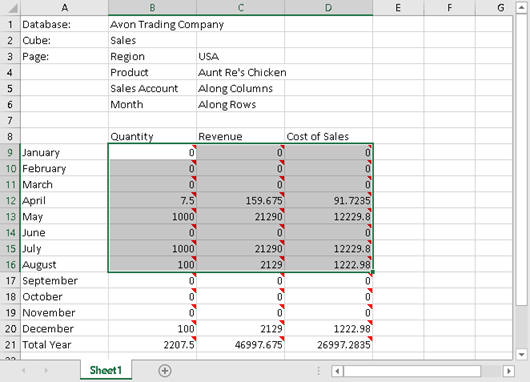14. Press F9 to recalculate.

Note that other cells are now populated with a range of like database references, and they return Fact Data that exists in their coordinates in the PowerOLAP database.

Edit Formula Text

PowerOLAP® allows you to modify database reference formula arguments. Using the Edit Formula Text command found on the PowerOLAP menu, you can change a single cell reference, or change the entire database reference.

1.   Open an Excel worksheet connected to a PowerOLAP database
(you can use the same worksheet from the previous example).

2.   Click on a cell containing a database reference formula,
e.g., cell B9 in the example worksheet.

3.   From the Excel ribbon, go to Add-Ins tab, select PowerOLAP and Edit Formula menu command.
The Edit PowerOLAP Formula Wizard is opened, with the database reference cell (B1) active for editing:TipSelecting the PowerOLAP Edit Formula button,, will also open the Edit PowerOLAP Formula Wizard.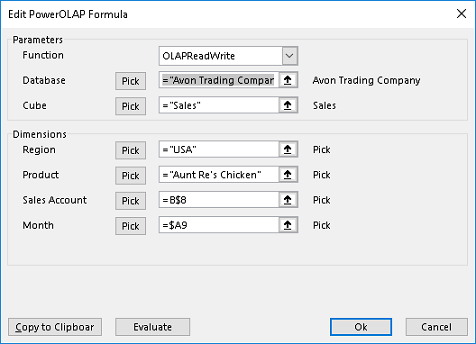As in the previous exercise, the dialog box displays the formula that references a single data point in a PowerOLAP database. In this dialog box, you can manually change the formula or use the Pick boxes to specify a different data point.

4.   Make changes to the formula as desired.

5.   Click OK to update and return to Excel.